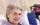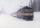Wood

Peter chops wood. If they cut down a half hours a day he ended work in 9 days. But Peter needs to end work 3 days earlier. How many minutes a day should cut down?

Result

x =  45 m

Solution:

9*30=(9-3)*x

6x = 270

x = 45

Calculated by our simple equation calculator.

Leave us a comment of this math problem and its solution (i.e. if it is still somewhat unclear...):Be the first to comment!To solve this verbal math problem are needed these knowledge from mathematics:

Do you have a linear equation or system of equations and looking for its solution? Or do you have quadratic equation?

Next similar examples:

1. HoursThe lesson lasts 45 minutes. For the week, students have 18 lesson hours. How many are the actual hours?
2. Six te 2If 3t-7=5t, then 6t=
3. Find xSolve: if 2(x-1)=14, then x= (solve an equation with one unknown)
4. FamilyMom is 39 years old. Karl is eight years and Paul 4 years. For many years mother would old just like two children together?
5. Addition of Roman numbersAdded together and write as decimal number: LXVII + MLXIV
6. Equation?
7. Bed timeTiffany was 5 years old; her week night bedtime grew by ¼ hour each year. If, at age 18, her curfew time is 11pm, what was her bed time when she was 5 years old?
8. QuizTested student answered correctly on a 4/ 8 questions. Wrong answers was 16. How many questions answered?
9. Negative in equation2x + 3 + 7x = – 24, what is the value of x?
10. One-thirdA one-third of unknown number is equal to five times as great as the difference of the same unknown number and number 28. Determine the unknown number.
11. Simple equationSolve the following simple equation: 2. (4x + 3) = 2-5. (1-x)
12. TrainThe train passes part of the line for 95 minutes at speed 75 km/h. What speed would have to go in order to shorten the driving time of 20 minutes?
13. EquationSolve the equation: 1/2-2/8 = 1/10; Write the result as a decimal number.
14. Simple equation 8Solve the following equation: 36=-(1+7x)-6(-7-x)
15. Simple equationSolve for x: 3(x + 2) = x - 18
16. Unknown numberIdentify unknown number which 1/5 is 40 greater than one tenth of that number.
17. How oldThe student who asked how many years he answered: "After 10 years I will be twice as old than as I was four years ago. How old is student?